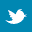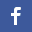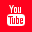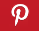Completely Solved C, C++ Programs Assignment.Quick Search Database Videos Tutorials Ebooks Forums FAQ Aboutus Household Industrial Manufacturing Service Shopping Transportation### Write a program to display n-th Fibonacci number

 Filed Under: C Assignments

PROBLEM STATEMENT:
Write a program to display n-th Fibonacci number.

THEORY:
In mathematics the Fibonacci number are the numbers in the following integer sequence.
0,1,1,2,3,5,8,13,21……
By definition, the first two Fibonacci numbers are 0 and 1, and each subsequent number is the sum of the previous two.
In mathematical terms, the sequence Fn of Fibonacci numbers is defined by the recurrence relation.
Fn = Fn-1 + Fn-2
with seed value F0 = 0 and F1 = 1

ALGORITHM:
/*algoithm to display nth fibonacci number*/

procedure fibonacci(n)
Begin fibonacci
fibo1<-0
fibo2<-1

for i=2 to n-1

fibo3<-fibo1+fibo2
fibo1<-fibo2
fibo2<-fibo3
i<-i+1
end for
return (fibo3)
End fibonacci

PROGRAM LISTING:
/*program to display nth fibonacci number*/

#include<stdio.h>
main()
{
int fibo(int);/*function to calculate n th fibonacci number*/
int n,result;

printf("n please enter the number upto which fibonacci series should be continued=");
scanf("%d",&n);

result=fibo(n);/*result of the fibonacci series*/

printf("n result of the fibonacci series=%d",result);
}

int fibo(int n)
{
int fibo1=0,fibo2=1,fibo3,i;
for(i=2;i<n;i++)
{
fibo3=fibo1+fibo2;
fibo1=fibo2;
fibo2=fibo3;
}
return(fibo3);

}

OUTPUT:
Please enter the number up to which Fibonacci series should be continued=5
Result of the Fibonacci series=3

DISCUSSIONS:
• Fibonacci numbers can be calculated by adding previous two numbers.
• Fibonacci numbers can be calculated by using recursive method also.
Fibonacci using recursions:-
int fibo(int n)
{
if(n==0 && n==1)
return;
else
return(fibo(n-1)+fibo(n-2)) ;
}Back to main directory:Software Practical